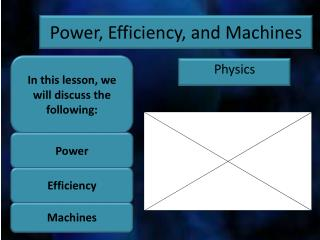Download PresentationPower, Efficiency, and Machines

# Power, Efficiency, and Machines

Download Presentation## Power, Efficiency, and Machines

- - - - - - - - - - - - - - - - - - - - - - - - - - - E N D - - - - - - - - - - - - - - - - - - - - - - - - - - -
##### Presentation Transcript

1. In this lesson, we will discuss the following: Power, Efficiency, and Machines Power Physics Efficiency Machines

2. Definition of Power Power is defined as how quickly work is done.

3. Abbreviation for Power Power is abbreviated P. P

4. Formula for Power Power is equal to work divided by time. • And, work is equal to change in energy.

5. SI Units for Power The SI unit for power is the Watt (W). 1 Joule 1 second

6. Power is a Scalar Quantity Power, like work and energy, is a scalar quantity. In other words, power has a magnitude and a unit. Power does not have a direction.

7. Using Power, Work, and Time in Calculations Problem: What is the power of a light bulb which does 216,000 Joules of work in one hour? Solution:

8. Using Power, Work, and Time in Calculations Click HERE if the page at the right does not open.

9. Definition of Efficiency Efficiency is defined as the percentage of useful energy you get out of a machine.

10. Abbreviation for Efficiency Efficiency is abbreviated ε. Lower-case epsilon.

11. Formula for Efficiency The efficiency of a machine is equal to useful work you get out of the machine divided by the work put into the machine times 100%.

12. Formula for Efficiency Or, the efficiency of a machine is equal to useful energy you get out of the machine divided by the energy put into the machine times 100%.

13. Efficiency is Dimensionless Efficiency is a dimensionless quantity. In other words, efficiency has no unit(s), unless you call percent a unit. I don’t.

14. Efficiency is a Scalar Quantity Efficiency is a scalar quantity. In other words, it has no direction.

15. Using Efficiency in Calculations Problem: What is the efficiency of a car which puts out 300 J of energy for every 1000 J of energy it uses? Solution:

16. Using Efficiency in Calculations Click HERE if the web page on the right does not open.

17. Machines and the Law of Conservation of Energy All machines must follow the Law of Conservation of Energy. In other words, the energythat is put into a machine is always at least as large as the useful energy that is taken out of the machine. In addition, the work that is put into any machine is always at least as large as the useful work that is taken out of the machine.

18. Machines and the Law of Conservation of Energy If a machine is 100% efficient, the work put into a machine is exactly equal to the useful work that is taken out of a machine. Workin = Workout (Forcein) x (Distancein) = (Forceout) x (Distanceout) Then you must exert that small force over a long distance… If you want to put a small force into a machine… And if you want to get out a larger force… And only get a small distance out.

19. Machines and the Law of Conservation of Energy Workin = Workout (Forcein) x (Distancein) = (Forceout) x (Distanceout) A car jack follows the Law of Conservation of Energy. A small force put into the jack exerted over a long distance results in a force large enough to pick up a car but over a very small distance.

20. Six Examples of Simple Machines All of these machines follow the Law of Conservation of Energy! Below you will find six examples of simple machines: • Lever • Inclined Plane • Wheel and Axle • Screw • Wedge • Pulley Click HERE if the web page at the right does not open.

21. Six Examples of Simple Machines Click HERE if the web page at the right does not open.

22. Six Examples of Simple Machines Click HERE if the web page at the right does not open.